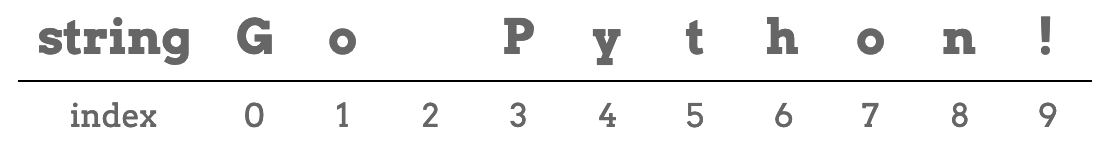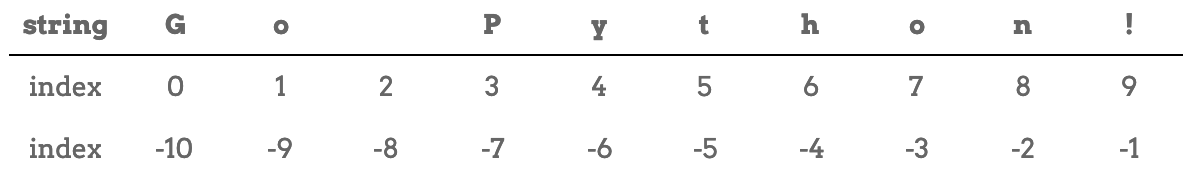# 7.2. Bracket Notation¶

Strings are ordered collections of characters, and this gives us a nice mental model for how they are put together. Each character has its own specific location within a string. We call this location the index.

Consider the string `'Go Python!'` The first character, `'G'`, has an index value of 0, the first `'o'` has index 1, the space `' '` has index 2, and so on. Index values are integers representing the position of a character within a given string.The index values of a string.

Note that this is another example of zero-based indexing. The count begins with the value 0.

## 7.2.1. Access One Character¶

Bracket notation is the special syntax that allows us to access the single characters that make up a string. To access a character, we use the syntax:

```some_string[index]
```

where `index` is the position of the character we want.

The expression `'school'` selects the character at index `2` and creates a new string containing just that one character.

With zero-based indexing, the letter at index 0 of `'school'` is `'s'`. So at position  we have the letter `'h'`.

Try It!

Predict which letters will be printed to the console. Check your answers by clicking Run. Remember that with zero-based indexing, the first character always has an index value of `0`.

Now try the following:

1. Change the index values in lines 3 and 5 to see how they affect the output.

2. Enter `40` into the brackets in line 3. What happens when you use an index value that is larger than the length of the string?

3. Replace `40` inside the brackets with a negative number, like `-1`. What happens?

In step 2 above, `this_string` causes an index out of range error. This happens anytime we try to reference an index location that does not exist in the string.

We will discuss what a negative index value returns in step 3 shortly.

### 7.2.1.1. Expressions for `index`¶

If we want to access the last character in a string, we need to know its index value. How can we find this number without having to count all of the characters?

An index value must either be an integer—like 0, 1, 2, etc.—or an expression that evaluates to an integer.

Recall that we can use the `len()` function to return the number of characters in a string.

Example

 ```1 2 3``` ```this_string = 'Zero-based indexing!' print(len(this_string)) ```

Console Output

```20
```

`len(this_string)` evaluates to 20, and that value gets printed to the console.

In the Try It example above, replace `print(this_string)` with `print(this_string[len(this_string)])`.

Wait…what? We got an index out of range error, but we KNOW that `this_string` is 20 characters long!

The reason is, once again, zero-based indexing. Since we start counting index values at `0`, the 20th character has an index value of `19`.

Tip

We can access the last character of the string and avoid the out of range error by using:

```print(this_string[len(this_string) - 1])
```

The expression `len(this_string) - 1` evaluates to `19`, and `this_string` is the last character (`'!'`).

## 7.2.2. Negative Index Values¶

Consider the string `'Go Python!'` again. From left to right, the characters take the index values 0 - 9.

Python also allows us to use index values that move from the end of the string to the beginning (right to left). In this case, the index values are negative integers.The positive and negative index values of a string.

Note that when we move from right to left, the index values start with `-1`.

### 7.2.2.1. Try It!¶

1. Use a negative index to print the character `'x'` from the string `Zero-based indexing!`

2. What is the smallest negative number that gives an index out of range error? In this case, “smallest” means “closest to zero”.

3. `this_string[len(this_string)]` throws an error. What about `this_string[-len(this_string)]`?

Question

If `phrase = 'Code for fun'`, then `phrase` evaluates to:

1. 'o'
2. 'd'
3. 'for'
4. 'fun'

Question

Which of the following returns `True` given `my_str = 'index'`? Choose ALL correct answers.

1. my_str == 'n'
2. my_str == 'x'
3. my_str == ' '
4. my_str == 'i'

Question

What is printed by the following code?

 ```1 2``` ```phrase = "Python rocks!" print(phrase[len(phrase) - 9]) ```
1. 'o'
2. 't'
3. 'n'
4. 'c'

Question

Given `my_str = 'ABC DEF GHI'`, which of the following expressions returns `'F'`?

1. my_str[-3]
2. my_str[-4]
3. my_str[-5]
4. my_str[-6]